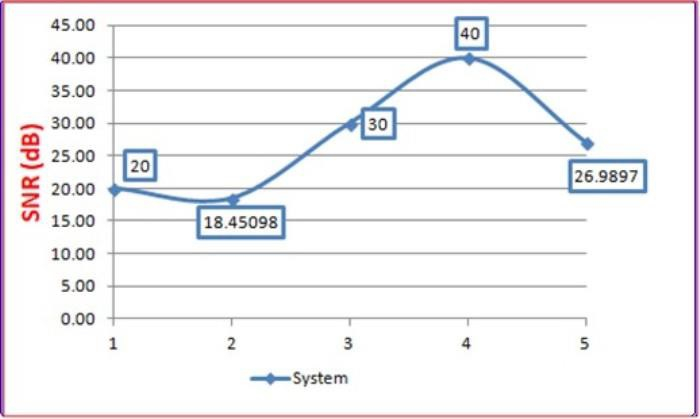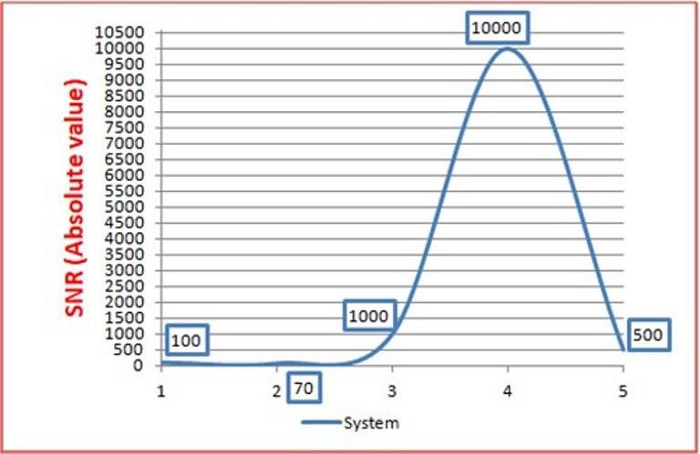# What is Signal to Noise Ratio?

The ‘Signal-to-Noise’ ratio or, SNR (in short), is a metric that describes the signal performance in the presence of wireless channel noise (interference). In the linear scale, the SNR is the ratio of the signal power to the noise power. The wireless channel is never noise-free.

There always exist noise floors that the signal must combat to reach the receiver successfully. At the receiver, we, therefore, not only receive the transmitted signal. We have a noisy or, noise added version of the transmitted signal.

Below is an example of SNR## Expression for SNR

$$SNR=\frac{Signal\:Power}{Noise\:Power}$$

$$SNR(dB)=10log_{10}(\frac{Signal\:Power}{Noise\:Power})$$

## Key Properties of SNR

• Signal to Noise ratio in short is called as SNR and is used to measure signal performance of wireless channel noise.

• Wireless channel noise is never noise-free.

• In linear scale, SNR is the ratio of signal power to noise power.

## What Information does SNR Give Us?

When the signal power drops below a certain threshold, it cannot be detected and decoded faithfully by the receiver. This is what SNR informs us. It tells us the strength of the signal when compared to the channel noise. A positive SNR indicates that the signal power is greater than the noise power while a negative SNR indicates the opposite.

It is often a common practice to express the SNR in logarithm-based decibel (dB) scale. The SNR is essentially unit less when expressed both on the linear scale (absolute value) and on the dB scale.

The wireless channel is noisy and also, different channels have different noise levels (and noise profiles/ probability density functions (PDFs)). Therefore, whenever a comparison is made between the SNR of different systems, it is difficult to have a convenient single graphical representation of the SNRs being compared.

EXAMPLE

Let the measured SNRs of four different systems be 100, 500, 1000 and 5000

Let us see the expression of SNR below −

$$SNR(dB)=10log_{10}(\frac{S_{p}}{N_{p}})$$

Where SP denotes signal power and NPdenotes noise power.

## Expressing SNR in Linear And Absolute Scale

S/MSNR(Abs)SNR(dB)
110020
27018.45098
3100030
41000040
550026.9897## Understanding The SNR Magnitude

Let us see what an SNR of 25 dB mean for a wireless channel implies.

$$25dB=10log_{10}(\frac{S_{p}}{N_{p}})$$

$$log_{10}(\frac{S_{p}}{N_{p}})=2.5$$

$$(\frac{S_{p}}{N_{p}})=10^{2.5}\approx\:316.22$$

So, we can observe that the ratio of signal to noise power is 31.622 and this indicates that the signal power is nearly 32 times stronger than the noise power and hence the signal doesn’t get buried under the noise.

## Ideal Value of SNR

There is no fixed value, but it varies depending on channel environments. If the channel is too noisy, a high SNR is desired.

## 0 dB SNR

The SNR is 0 dB when the signal power and noise power equal each other. This is a worse case. Unfortunately, the receiver can’t detect and decode the signal. Let us see look at this scenario below −

$$SNR(dB)=10\:log_{10}(\frac{S_{p}}{N_{p}})=10\:log_{10}(\frac{N_{p}}{N_{p}})=0$$

The SNR is 0 dB when the signal power equals the noise power. Any SNR value including 0 dB and below this value is considered worse.